Ch 2. Vectors Multimedia Engineering Statics Scalars & Vectors 2-DVectors 3-DVectors DotProducts
 Chapter 1. Basics 2. Vectors 3. Forces 4. Moments 5. Rigid Bodies 6. Structures 7. Centroids/Inertia 8. Internal Loads 9. Friction 10. Work & Energy Appendix Basic Math Units Sections Search eBooks Dynamics Fluids Math Mechanics Statics Thermodynamics Author(s): Kurt Gramoll ©Kurt GramollSTATICS - THEORY Vector Components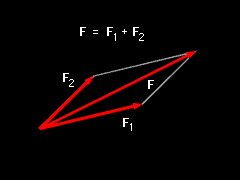Vector Components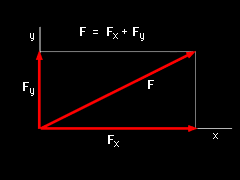X-Y Axis Orientation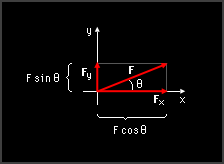Vector Components Diagram In the previous section, vectors were described using its magnitude and direction. This makes mathematical operations difficult (cannot simply add angles). To help solve this problem, vectors are usually split into components. For example, if two vectors, F1 and F2, give the vector F when added together, then F1 and F2 are said to be components of the vector F:      F = F1 + F2 If the vectors F1 and F2 are perpendicular to each other, they are called rectangular, or Cartesian, components. If the x-y axis is oriented along these components, they are labeled Fx and Fy respectively:      F = Fx + Fy If two vectors, i and j, have a magnitude of one and are in the x and y direction respectively, then F can be written as      F = Fxi + Fyj The vectors i and j are called Cartesian unit vectors, and Fx and Fy are the scalar components of the vector F. This configuration is the most common to describe a vector and allows vectors to be added and subtracted quickly and easily. If the angle θ is measured from the x axis counterclockwise, then the scalar components are      Fx = F cosθ      Fy = F sinθ General Unit Vectors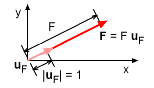General Unit Vector uF Unit vectors in the x and y directions (i and j) where used the above paragraphs, but unit vectors can also be used in any direction. Unit vectors give direction where magnitude gives the length of the vector. Unit vectors are defined as      uF = F/F and have a magnitude of 1. Vector Addition and Subtraction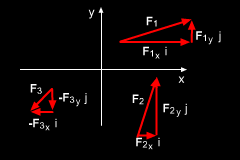Addition of Multiple Vector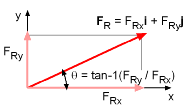Vector Angle Relationship When a set of vectors are described using Cartesian unit vectors (i and j), then the resultant vector is just the addition (or subtraction) each components, giving      FR = F1 + F2 +... = ΣFxi + ΣFyj        where ΣFx = F1x + F2x +...                  ΣFy = F1y + F2y +... Note, Fn-x and Fn-y are the components of each individual vector where n is the numbering of each vector being added. The magnitude and direction of the resultant vector, FR, can be determined just like any individual vector by,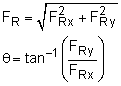Practice Homework and Test problems now available in the 'Eng Statics' mobile app
Includes over 500 problems with complete detailed solutions.
Available now at the Google Play Store and Apple App Store.